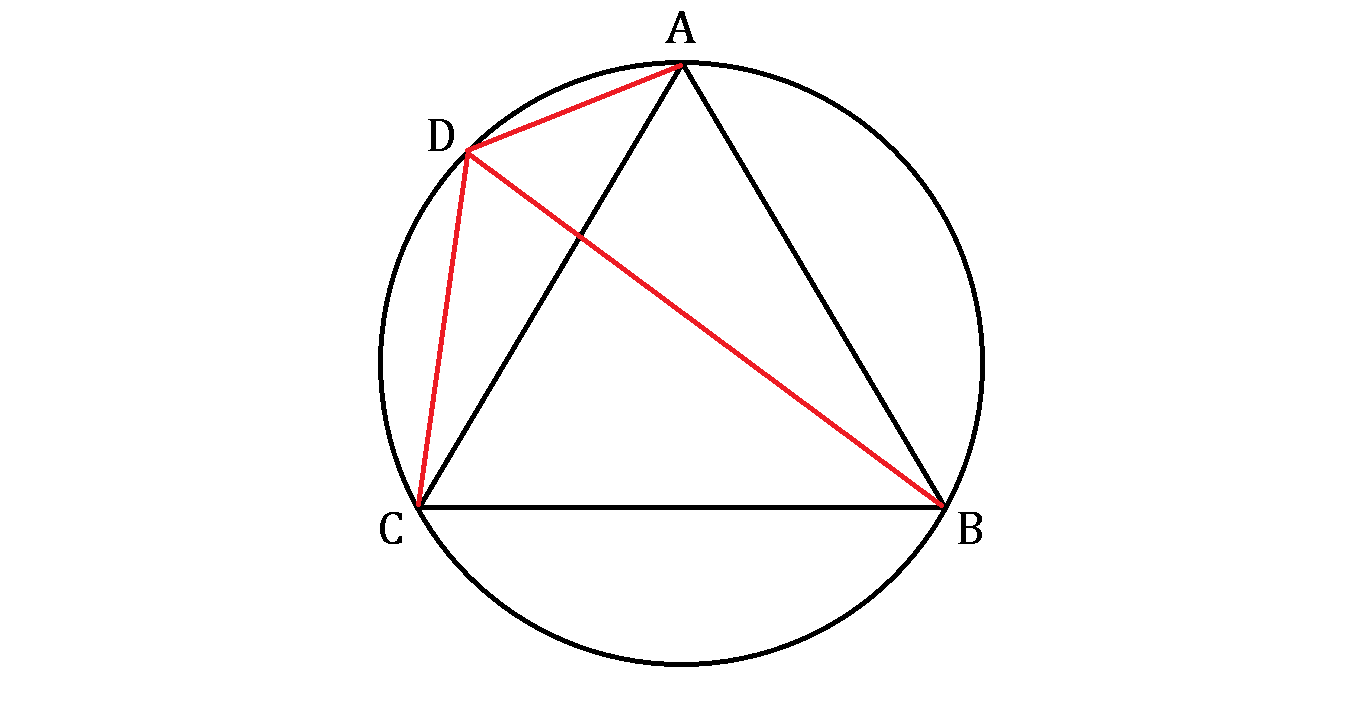# Ptolemy's Riddle II

Once upon a time, Ptolemy let his pupil draw an equilateral triangle $ABC$ inscribed in a circle before the great mathematician depicted point $D$ and joined the red lines with other vertices, as shown below.

Ptolemy: Dost thou see that all the red lines have the lengths in whole integers?
Pupil: Indeed, master! Such an extraordinary point!
Ptolemy: Now if the equilateral triangle has a side length of 13, what is the sum of the three red lengths combined?×